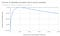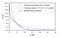# Impermanent Loss (how to not rope after providing liquidity)

To kick this Medium channel off I would do a quick write-up for everyone on how impermanent loss works in the DeFi world so you don’t lose money to the volatile price changes we all love (seriously we are trading crypto).

# Why do people provide liquidity, why do we need it?

In business, economics or investment, market liquidity is a market’s feature whereby an individual or firm can quickly purchase or sell an asset without causing a drastic change in the asset’s price.

Aight so I learned it is better to provide the definition of liquidity first before talking vaguely about it, so there you have it, anyways…

So the idea is simple, let us examine it:

Take the Ethereum mainnet as an example, there are hundreds of different coins on the mainnet that people want to buy (there are thousands but the “important” ones are in the 100’s). But, the coins usually aren’t available on large centralized exchanges (cexes) when they are first released, instead, they usually appear first on decentralized exchanges (dexes) like Uniswap, Sushiswap, 1inch, where they can be swapped for.

But how do they appear? Well, people usually somehow (how they do it is not important) acquire them, and then just offer them to the dex with another coin (ERC 20 token, whatever) for trading (“providing liquidity”). For example, if the A-token just appeared on the market and you want to provide liquidity, you could get A and then create a pool that is made of an A — ETH pair. You would then deposit an amount of Ethereum and A, that is equal to each other in price. Let paint demonstrate what I mean:

An example of a pair on UNISWAP: https://info.uniswap.org/pair/0xb4e16d0168e52d35cacd2c6185b44281ec28c9dc

So now, people can trade A for ETH or ETH for A on Uniswap. The provider also doesn’t do this for free, the dex charges fees on swaps, which are distributed to the LPs.

The more LPs there are, the more coins there are, the more can people trade safely on a dex and avoid having to deal with other stuff.

That is basically the idea behind providing liquidity.

# Road to IL: How do the pools work specifically?

Alright, so, we saw that we can deposit pools and get fees, but how does this work specifically? It works through math. I will now attempt to shove this into your face in a bunch of simple concepts:

So first of all. Let’s take two tokens A and B. Let us say that B is worth two A. So if you want to start with a simple ratio of the tokens inside the pool, we can just set the starting to be B = 2A. And you can scale that as much as you want.

So let’s imagine (majin) a pool that has 200 A and 100 B. The ratio is perfect.

Now, if you want to provide more liquidity, you have to first deposit the tokens in the same ratio, so, let’s say you’re giving 10 B, you also have to add 20 A.

Aight, so the pool now has 220 A and 110 B.

How much liquidity do you own in the pool now? Easy. Just take either A or B. Just divide the amount you added, by the total amount of that token in the pool. So, for example for B, 10/110 = 0,09090909 periodic. Let’s round that to 0.091 which is 9.1%. You now own 9.1% liquidity in the pool.

This automatically means you receive the following amount of fees:

0.091 * total_fees = your_fee

Alright, but how does swapping even work, like how do the maths work when the tokens get actually used?

Easy.

We now have 220A in the pool and 110 B. Currently 2A=B (as in, how much you should theoretically get).

Aight, now I come and swap exactly 53 B for 106 A, since B is obviously two A. So what now, how do the pool dynamics work? What is the price after swapping?

Well, firstly, you have to understand that there is a lot of things that influence the price of some cryptocurrency. When people swap tokens, on which dex people swap tokens, how much liquidity there is on the dex, how the dex works, and so on…

As such, right now, there is a lot of different models of how the price is determined in pools. Uniswap has changed details of it between its two versions for example (as of the current time of writing).

Secondly, the first was a trick question. First of all, the pool doesn’t determine the next price after swapping but determines the price FOR the swap, which means, the above COULD have been a valid swap, but only if the system determined it according to its equations. I will now go over one of the simplest ones, the one that is being used on Uniswap right now, in a general overview.

How this works:

Let’s say that NA is the number of the A tokens in the pool. Let’s says that NB is the number of the B tokens in the pool.

On Uniswap, simplified, the tokens in the pool have to satisfy the following equation (ignoring fees):

NA * NB = K

That means that for our example, we would have for the first situation:

1. NA = 220, NB = 110, K = 24200.
2. I now want to sell 53 B. Alright so what is the price of B?
3. NB = 163, so NA = K/NB = 148,46626, something like that
4. So the price of B = (220–148,46626)/53 A= 1,349693207 A.

So, the price obviously changes, so how does this look graphically? An excel plot:

The graphs are symmetrical and have the same shape for both the price and the token distribution (to clear up confusion). A and B are readily interchangeable on these graphs.

# Impermanent Loss

Aight.

Now impermanent loss is also simple once you understand the above.

Just consider the following:

1. At the start, there was NA = 220 and NB = 110 tokens in the pool. Let us assume for simplicity you own 20% of the liquidity, so 22 B and 44 A. Which is exactly 88 A.
2. After the swap, there is NB = 163 and NA = 148,46626 in the pool, so you own 32,6 B and 29,693252 A, which is about 65,4845 A (since the B price is now 163/148,46626).
3. Your IL at this point: a little less than 25,586%.From real trial and error, we get this graph, taken from UNISWAP

There are also other DAO’s that use different equations which result in different IL movements:

From the Curve Whitepaper:Plots of different equations, note that an equation of the form x+y = const is not feasible in a real market

# Extras

Use https://defiyield.info/ or something similar to help you out. https://harvest.finance/ doesn’t take gas fees once you deposit for intra-movements that are used to optimize APY.

Don’t provide liq with shitcoins that you think are about to moon unless you know what you are doing, gas fees will eat you up, and IL will possibly allow it.

That would be all.

ADD: if I missed something msg me